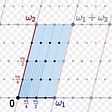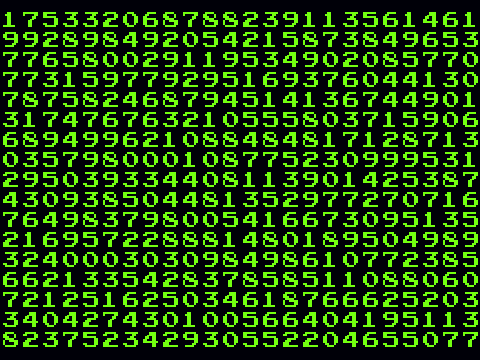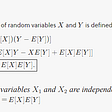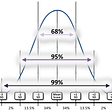# Naive Bayes: Implementation from scratch.

• Naive means that the features are independent of each other and the inference of anything will be contributed of all features.
• Bayes is from the Bayes theorem .
• P(A|B): Posterior probability.
• P(A): Prior probability.
• P(B|A): Likelihood.
• P(B): Evidence.
• prior_probability(): Probability of hypothesis before observing the evidence.
• statistics(): This calculates mean, variance which needed for gaussian distribution for each column and convert to numpy array
• gaussian_density(): This is the Gaussian Distribution here u signifies the mean, sigma is the standard deviation and sigma² is the variance statistics() function feeds input to it.
• posterior_probability(): This calculates P(A) Probability of hypothesis A on the observed event B.
• fit(): This functions fits our Naive Bayes Model.
• predict(): This function provides predictions.
• accuracy():

--

--

--

## More from Akshar Rastogi

Love podcasts or audiobooks? Learn on the go with our new app.

## Are you calculating leap years incorrectly?## Doud’s Method: Using the theory of elliptic curves over C to do something useful over Q## How Computers Make Random Numbers## Co-Variance in Words## Be aware: Slack variables are not decision variables.## Simplification = destruction? ♻️On the path of optimization and simplification## 3 Ways to Find the Area Under a Curve (and why you would want to do that)## R Programming: Distributions and Central Limit Theorem## Train a custom dataset for object detection using Haar Cascade in Windows## Traffic Sign Classifier## Car Race using pygame in python## What is Java Virtual Machine(JVM)?